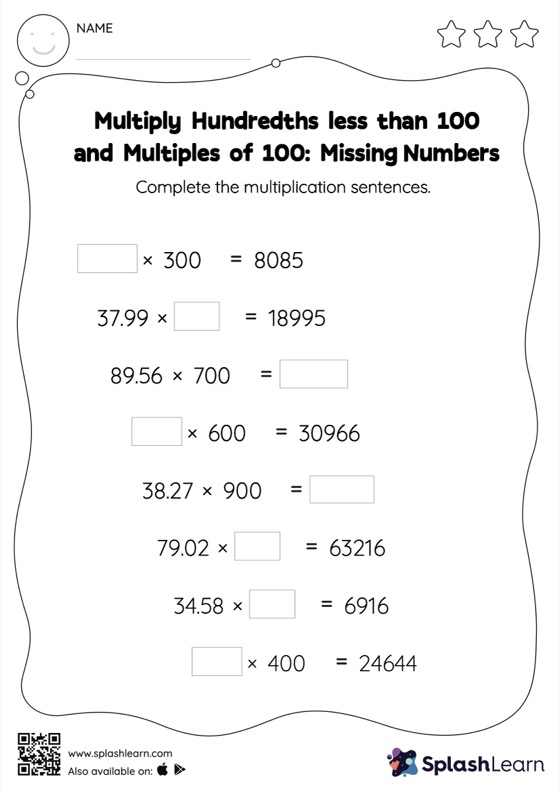# Multiply Hundredths less than 100 and Multiples of 100: Missing Numbers Worksheet

Home > Multiply Hundredths less than 100 and Multiples of 100: Missing NumbersThis worksheet strengthens students' accuracy and strategic flexibility while they multiply hundredths less than 100 and multiples of 100. Students understand that multiplying a decimal by a number that ends in zeros causes the decimal point to be moved to the right by the same number of places as the multiplier's number of zeros. This understanding helps them find the missing number in multiply hundredths less than 100 and multiples of 100 worksheet. In each problem, the numbers are laid out in the horizontal format. Students should try to use different strategies involving composing and decomposing numbers to solve these problems. This will help them develop flexibility and fluency.